GFG App
Open AppBrowser
Continue

# Regular grammar (Model regular grammars )

Prerequisites: Chomsky hierarchy

### Type-3 grammar/regular grammar:

Regular grammar generates regular language. They have a single non-terminal on the left-hand side and a right-hand side consisting of a single terminal or single terminal followed by a non-terminal.

The productions must be in the form:

```A ⇢ xB
A ⇢ x
A ⇢ Bx
where A, B ∈ Variable(V) and x ∈ T*  i.e. string of terminals.```

### Types of regular grammar:

• Left Linear grammar(LLG)
• Right linear grammar(RLG)

1. Left linear grammar(LLG):
In LLG, the productions are in the form if all the productions are of the form

```A ⇢ Bx
A ⇢ x
where A,B ∈ V and x ∈ T*```

2. Right linear grammar(RLG):
In RLG, the productions are in the form if all the productions are of the form

```A ⇢ xB
A ⇢ x
where A,B  ∈ V and x ∈ T*```

The language generated by type-3 grammar is a regular language, for which a FA can be designed. The FA can also be converted into type-3 grammar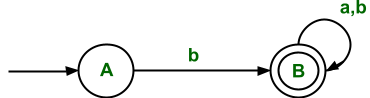```∑  = {a,b}
Initial state(q0) = A
Final state(F)  = B```

The RLG corresponding to FA is

```A ⇢ bB
B ⇢  ∈/aB/bB ```

The above grammar is RLG, which can be written directly through FA.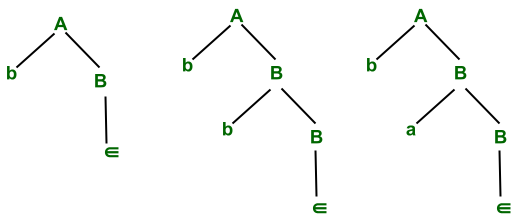This grammar derives strings that are stated with B

The above RLG can derive strings that start with b and after that any input symbol(i.e. ∑ ={a, b} can be accepted).

```The regular language corresponding to RLG is
L= {b, ba, bb, baa, bab ,bba,bbb ..... }```

If we reverse the above production of the above RLG, then we get

```A ⇢ Bb
B ⇢  ∈/Ba/Bb
It derives the language that contains all the strings which end with b.
i.e. L' = {b, bb, ab, aab, bab, abb, bbb .....}```

So we can conclude that if we have FA that represents language L and if we convert it, into RLG, which again represents
language L, but after reversing RLG we get LLG which represents language L'(i.e. reverse of L).

For converting the RLG into LLG for language L, the following procedure needs to be followed:

```Step 1: Reverse the FA for language L
Step 2: Write the RLG for it.
Step 3: Reverse the right linear grammar.
after this we get the grammar that generates the language that represents the LLG for the same language L.```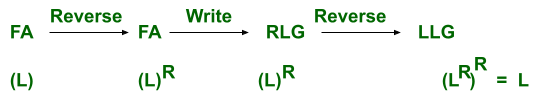This represents the same procedure as above for converting RLG to LLG

Here L is a language for FA and LR is a reversal of the language L.
Example:
The above FA represents language L(i.e. set of all strings over input symbols a and b which start with b).
We are converting it into LLG.

Step1: The reversal of FA is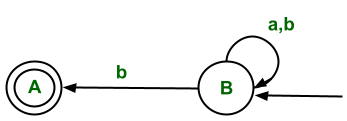The reversal of FA represents all strings starting with b.

Step 2: The corresponding RLG for this reversed FA is

```B ⇢ aB/bB/bA
A ⇢  ∈```

Step 3: The reversing the above RLG we get

```B ⇢ Ba/Bb/Ab
A ⇢  ∈```

So this is LLG for language L( which represents all strings that start with b).
L= {b, ba, bb, baa, bab ,bba, bbb ….. }

Conversion of RLG to FA:

• Start from the first production.
• From every left alphabet (or variable) go to the symbol followed by it.
• Start state: It will be the first production state.
• Final state: Take those states which end up with terminals without further non-terminals.

Example: The RLL grammar for Language(L), represents a set of all strings which end with 0.

```A ⇢ 0A/1B/0B
B ⇢ ∈```

So the FA for corresponding to RLG can be found out as

• For production A ⇢ 0A, this means after getting input symbol 0, the transition will remain in the same state.
• For production, A ⇢ 1B, this means after getting input symbol 1, the state transition will take place from State A to B.
• For production A ⇢ 0B, this means after getting input symbol 0, the state transition will take place from State A to B.
• For production B ⇢ ∈, this means there is no need for state transition. This means it would be the final state in the corresponding FA as RHS is terminal.

So the final NFA for the corresponding RLG is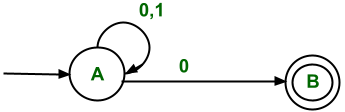Set of all strings that end with 0

### Conversion of LLG to FA: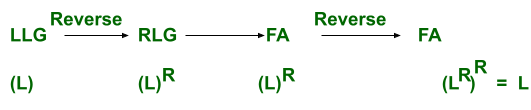Explanation: First convert the LLG which represents the Language(L) to RLG, which represents, the reversal of language L(i.e.LR) then design FA corresponding to it(i.e. FA for Language LR ). Then reverse the FA. Then the final FA is FA for language L).

Conversion of LLG to RLG: For example, the above grammar is taken which represents language L(i.e. set of all strings that start with b)
The LLG for this grammar is

```B ⇢ Ba/Bb/Ab
A ⇢  ∈```

Step 1: Convert the LLG into FA(i.e. the conversion procedure is the same as above)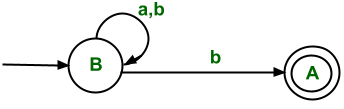Step 2: Reverse the FA(i.e. initial state is converted into final state and convert final state to initial state and  reverse all edges)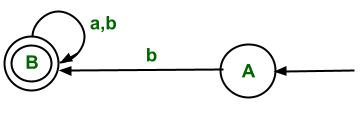Step 3: Write RLG corresponding to reversed FA.

```A ⇢  bB
B ⇢ aB/bB/∈```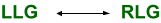They can be easily converted to other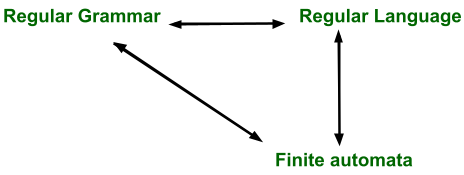All have the same power and can be converted to other

My Personal Notes arrow_drop_up